## Friday, 11 May 2012

### M2: Algebra and geometry Part 1

In HKCEE A-maths or HKDSE M2 paper, the topic "rate of change" as the application of differentiation always appear in the paper and sometimes it has been modelled into a geometric question like the velocity of a certain position of a pivot. What I want to illustrate is that geometric question can be modelled into a algebra question in many ways like vectors, coordinate geometry, and this time we can deal with calculus.

With the assistance of trigonometry we built up a strong relationship between segment lengths and angles, allowing us to relate different quantity, then the rate of change of a certain quantity can be transformed easily. We will demonstrate four questions, one unrelated to calculus, one A-math, one M2 and one Tokyo U questions, showing that differentiation can deal with meaningful conclusion instead of simply finding some derivatives.

Recall: Sine law and Cosine law.

Theorem. (Sine Law)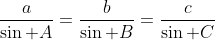Theorem. (Cosine Law)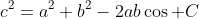Question 1. (Incircle) Let a,b,c be three sides of right-angled triangle ABC where c is the hyp. side. Show the incircle radius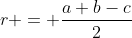Define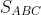be the area of the triangle ABC.

Now notice that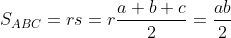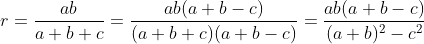Since it is right angled triangle,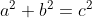, and therefore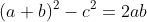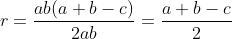Comparing with the x-y-z approach of incircle (Fact: AF = AG, CF = CE, BG = BE due to tangent properties or congruent triangle.) this is completed in a more algebrical way. (Using the x-y-z approach requires a cartesian plane which the right angled properties is shown in a geometrical simulation, but in this approach it is shown in a algebrical way).

Question 2. (Triangle on parabola) Let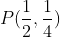be a point on the parabola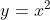,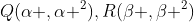are points on the same parabola such that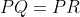. Let G be the centroid of the triangle PQR. Let angle QPR be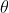.

Source: Tokyo University Entrance Exam (modified)

a) Find the locus of G in parametric form.

b) Find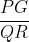in terms of the parameter used.

c) By (b) or otherwise, show that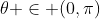.

By equating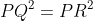,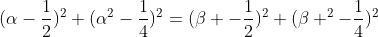, then we have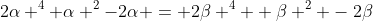.

Moving all terms to the left and do some factorization we have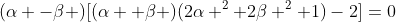. Due to the symmetricities of the parabola AND the insymmetricities between Q and R, we have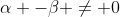, therefore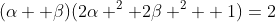.

Now it is a suitable time to parametize the equation. By simple trial and error, we know that the x-value of the locus tends to a certain finite value while the y-value converges. We want to make the point further from the origin for larger t. Therefore we let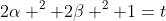, then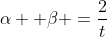.

Now we deal with the coordinate of G: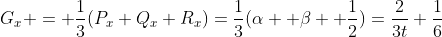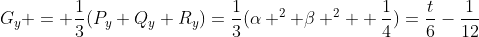By substituting the value of P we have the constrain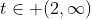, and that gives us the parametric equation desired.

In the above expression we can clearly see that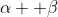tends to 1/6 as t gets larger (which is not zero and is a bit out of expectation). If you want the explicit equation it is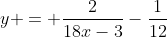where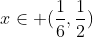.

Now when we proceed to part b, there are two quantity that we avoided in part a must be solved in this part, they are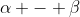and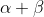. In DSE cirriculum we mainly derive the symmetric functions by Viete's theorem (or known as relationship between sum and products) and now, we start with two symmetric functionsand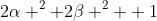.

Now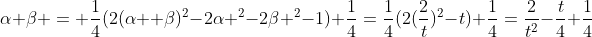. It is not suprising that it is negative when t is large because they are positive-negative pairs for enough big t.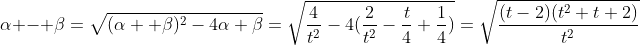which is a bit messy. After some simplification we can get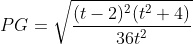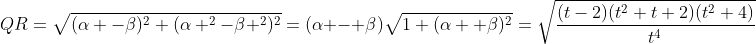Now we can compute the value questioned in part b.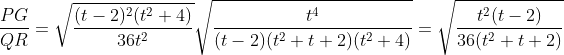For part c, we need a bit basic geometry knowledge. The median PX (where X on QR) is the altitude, angle bisector and perpendicular bisector of the triangle as well because PQ=PR. Also, since PX is the median, we have PG = 2/3 (PX) by properties of median. Therefore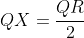,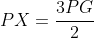. Consider trigonometric functinos,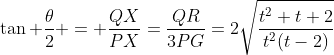. To make life easier we call if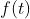.

The numerator of f is always positive, but the denominator tends to zero when it approach to 2 (which is the boundary case of the original figure), therefore f tends to infinity at t=2. Since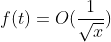, i.e., exist M and k so that for all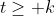we have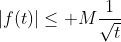, by sandwich or whatever it tends to zero. Therefore f tends to zero when t tends to infinity. The function is everywhere continous at defined t so that taking arctan gives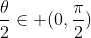which finishes the proof.

Of course there are some basic approach for part c (directly leaving part a and b unanswered) since the result is a bit stupid. We can do the approximation that when t is large enough,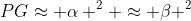and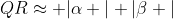which clearly leads to the limit when t tends to infinity. When t tends to 2, all points tends to point T, then QPR tends to be the tangent of the parabola, hence tending to 180 degrees.

I must admit that this is hardcore coordinate geometry but it's not the traditional pure maths questions (tangents involving conic sections), instead it emphasizes pretty much algebric skills in modelling a geometry problem.

Foods for thought. 1) (Rethinking parametization) When t is large enough, one suggests the approximation: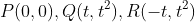on the same parabola. Then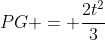and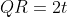, and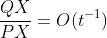instead of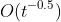! The above calculation is correct but the degree from approximation differs. What is happening?
2) (Generalization)
a) Instead of a fixed point P, find the locus of G if P is a varying point on the parabola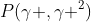, express your answer in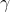and t.
b) Do the same generalization for part b and finish the proof in part c. (Well, calculuating the stuffs in part b is extremely complicated and is a bit pointless, but it is a good arithmetic exercise.)

Algebra and Geometry Part II